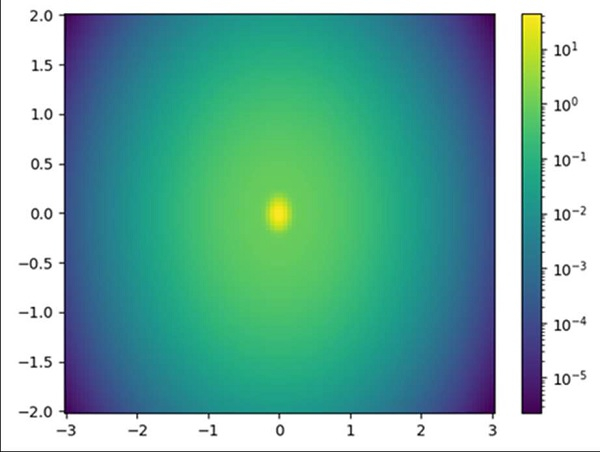# Defining the midpoint of a colormap in Matplotlib

Using plt.subplots(1, 1) method, we can create fig and axis. We can use fig.colorbar to make the color bar at the midpoint of the figure.

## Steps

• Using mgrid() method, nd_grid instance which returns an open multi-dimensional "meshgrid".

• Create Z1, Z2 and Z data.

• Create fig and ax variables using subplots method, where default nrows and ncols are 1, using subplots() method.

• Create a colorbar for a ScalarMappable instance, *mappable*, using colorbar() method.

• Using plt.show(), we can show the figure.

## Example

import numpy as np
import matplotlib.pyplot as plt
import matplotlib.colors as colors

N = 100
X, Y = np.mgrid[-3:3:complex(0, N), -2:2:complex(0, N)]

Z1 = np.exp(-(X)**2 - (Y)**2)
Z2 = np.exp(-(X * 10)**2 - (Y * 10)**2)
Z = Z1 + 50 * Z2

fig, ax = plt.subplots(1, 1)

fig.colorbar(ax.pcolor(X, Y, Z, norm=colors.LogNorm(vmin=Z.min(),
plt.show()